GATE  >  Test: Fluid Mechanics- 1

# Test: Fluid Mechanics- 1

Test Description

## 15 Questions MCQ Test GATE Civil Engineering (CE) 2023 Mock Test Series | Test: Fluid Mechanics- 1

Test: Fluid Mechanics- 1 for GATE 2023 is part of GATE Civil Engineering (CE) 2023 Mock Test Series preparation. The Test: Fluid Mechanics- 1 questions and answers have been prepared according to the GATE exam syllabus.The Test: Fluid Mechanics- 1 MCQs are made for GATE 2023 Exam. Find important definitions, questions, notes, meanings, examples, exercises, MCQs and online tests for Test: Fluid Mechanics- 1 below.
Solutions of Test: Fluid Mechanics- 1 questions in English are available as part of our GATE Civil Engineering (CE) 2023 Mock Test Series for GATE & Test: Fluid Mechanics- 1 solutions in Hindi for GATE Civil Engineering (CE) 2023 Mock Test Series course. Download more important topics, notes, lectures and mock test series for GATE Exam by signing up for free. Attempt Test: Fluid Mechanics- 1 | 15 questions in 45 minutes | Mock test for GATE preparation | Free important questions MCQ to study GATE Civil Engineering (CE) 2023 Mock Test Series for GATE Exam | Download free PDF with solutions
 1 Crore+ students have signed up on EduRev. Have you?
Test: Fluid Mechanics- 1 - Question 1

### If the velocity distribution over a plate is given by u = 2/3 y – y2 which v is the velocity in m/s at a distance y meter above the plate. The value of shear stress at y = 0 and y = 0.15m. Take dynamic viscosity of fluid as 8.63 poise.

Detailed Solution for Test: Fluid Mechanics- 1 - Question 1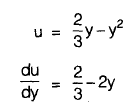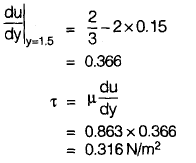Test: Fluid Mechanics- 1 - Question 2

### A globe valve is the most suitable for applications, in which

Detailed Solution for Test: Fluid Mechanics- 1 - Question 2

Butterfly valve: Regulates discharge
Ball valve: Maintains constant level in elevated tank
Foot valve: used in end of suction pipe
Globe valve: Similar to water tap, thus control fluid flow. Flow change its direction 90 degree twice to increase the head loss.
Pilot valve: Control high pressure and is used in emergency and safety controls.

Test: Fluid Mechanics- 1 - Question 3

### In the inclined manometer shown in figure below, the reservoir is large. Its surface may be assumed to remain at a fixed elevation. A is connected to a gas pipeline and the deflection noted on the inclined glass tube is 100 mm. Assuming θ = 30° and the manometric fluid as oil with specific gravity of 0.86, the pressure at A is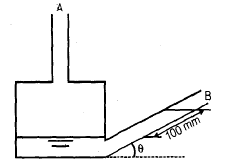Detailed Solution for Test: Fluid Mechanics- 1 - Question 3

Total pressure at A will be equal to the pressure at the surface of manometric fluid because above the surface, the gas pipeline is connected.
Pressure at A = 100 sin 30° mm of oil = 50 mm of oil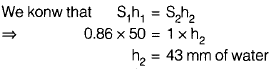Test: Fluid Mechanics- 1 - Question 4

If flow velocity in x and y directions are given by u = 2x + 3y and v = –2y. The circulation around the circle of radius 2 units will be

Detailed Solution for Test: Fluid Mechanics- 1 - Question 4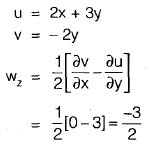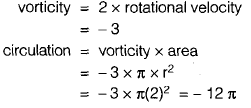Test: Fluid Mechanics- 1 - Question 5

For a two dimensional flow,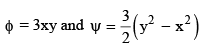Find the discharge passing between stream line passing through the points (1, 3) and (3, 3)

Detailed Solution for Test: Fluid Mechanics- 1 - Question 5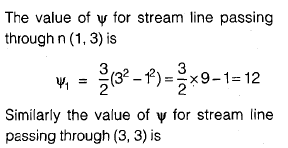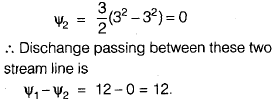Test: Fluid Mechanics- 1 - Question 6

Through a very narrow gap of height ‘h’ a thin plate of large extent is pulled at a velocity ‘v’. On upper side of the plate is oil is viscosity u1 and the other side of oil of viscosity u2 . At what distance from the lower surface the plate should be placed so that pull required to drag the plate is minimum

Detailed Solution for Test: Fluid Mechanics- 1 - Question 6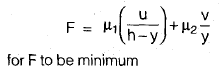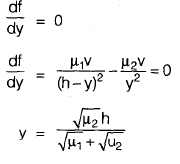Test: Fluid Mechanics- 1 - Question 7

The compartment B and C shown in the figure below are closed and filled with air the barometer reads 99.572 kN/m2. When the gauges A and D reads as indicated what should be the value of x for gauge E. (mercury is the monometric liquid in each U tube gauge)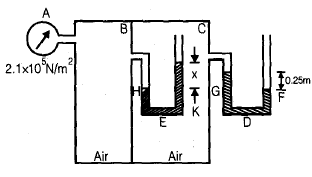Detailed Solution for Test: Fluid Mechanics- 1 - Question 7

If P is the pressure intensity of air in compartment C in terms of obsolute unit then

Test: Fluid Mechanics- 1 - Question 8

A cylinder 2.4 m diameter weights 2 kN and rests on the bottom of tank which is 1 m long. As shown in figure, water and oil are poured in to the left and right hand portions of the tank to depths 0.6 m and 1.2 m respectively. The magnitude of horizontal and vertical components of force which will keep the cylinder touching the tank at B will be respectively.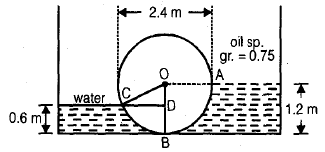Detailed Solution for Test: Fluid Mechanics- 1 - Question 8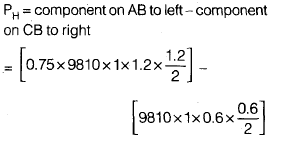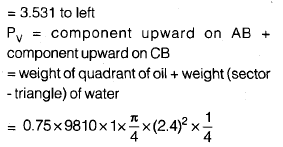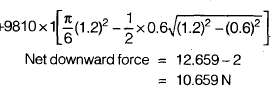Test: Fluid Mechanics- 1 - Question 9

Consider the following

The components of velocity u and v along X and Y directions in a 2D flow problem of an incompressible fluid are

1. u = x2 cos y; v = – 2x sin y
2. u = x + 2; v = 1 – y
3. u = xyt; v = x3 – y2t/2
4. u = ln x + y; v = xy – y/x

Which of these will satisfy the continuity equation?

Detailed Solution for Test: Fluid Mechanics- 1 - Question 9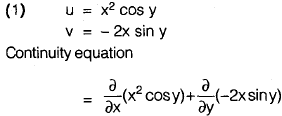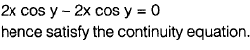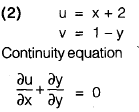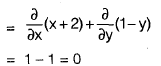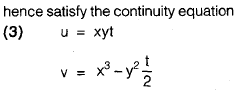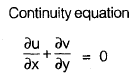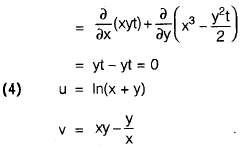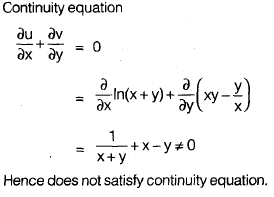Test: Fluid Mechanics- 1 - Question 10

A venturimeter has its axis vertical, the inlet and throat diameter being 150 mm and 75 mm respectively. The throat is 225 mm above inlet. Petrol of specific gravity 0.78 flows up at a rate of 0.029 m3/s. The pressure difference between the inlet and throat will be (cd = 0.96 for venturimeter)

Detailed Solution for Test: Fluid Mechanics- 1 - Question 10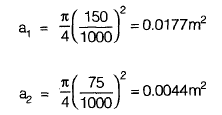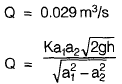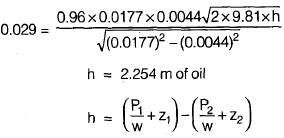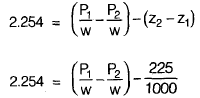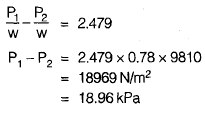Test: Fluid Mechanics- 1 - Question 11

Water flows from a 300 mm pipe. Two pitot tubes are installed in the pipe. One on the centre line and other 75 mm from the centre line. If velocities at the two points are 3m/s and 2m/s respectively calculate the reading on the differential manometer connected to the two tubes

Detailed Solution for Test: Fluid Mechanics- 1 - Question 11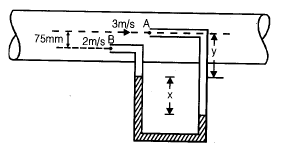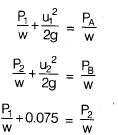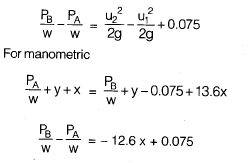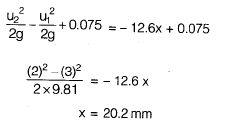Test: Fluid Mechanics- 1 - Question 12

A pipe ABC connecting two reservoirs is 80 mm in diameter from A to B the pipe is horizontal as shown in the figure and from B to C if falls by 3.5 meters the length AB and BC are 25 m and 15 m respectively. If the water in the presence at A is 4m above the centre line of pipe and at C 1 m above the centre line of pipe. Neglect the loss at bend but consider all other losses. Take f = 0.024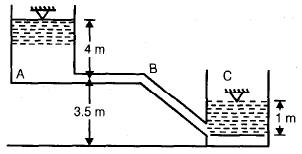Q.

Find the quantity of flow?

Detailed Solution for Test: Fluid Mechanics- 1 - Question 12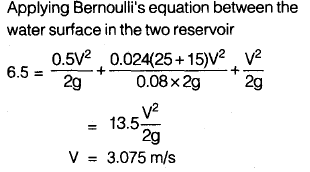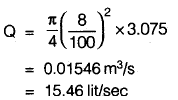Test: Fluid Mechanics- 1 - Question 13

A pipe ABC connecting two reservoirs is 80 mm in diameter from A to B the pipe is horizontal as shown in the figure and from B to C if falls by 3.5 meters the length AB and BC are 25 m and 15 m respectively. If the water in the presence at A is 4m above the centre line of pipe and at C 1 m above the centre line of pipe. Neglect the loss at bend but consider all other losses. Take f = 0.024Q.

The pressure head at B is

Detailed Solution for Test: Fluid Mechanics- 1 - Question 13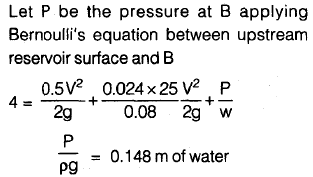Test: Fluid Mechanics- 1 - Question 14

Air flows over a flat plate 1 m long at a velocity of 6 m/s. The sheer stress at the middle of plate will be

[Take S = 1.226 kg/m3, v = 0.15× 10–4 m3/s (0.15 strokes) for air]

Detailed Solution for Test: Fluid Mechanics- 1 - Question 14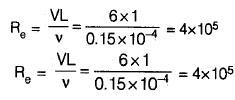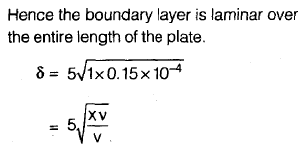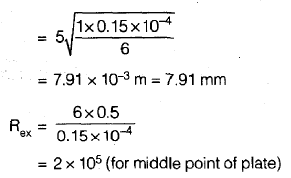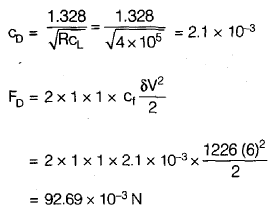Test: Fluid Mechanics- 1 - Question 15

Oil is pumped through a 7.5 cm diameter pipe, 1 km long. The dynamic viscosity of oil is 0.1 N s/m2. A power input of 5.4 kW with an overall efficiency of 60% at the pump is noted. The discharge through the pump is (specific gravity of oil is 0.9)

Detailed Solution for Test: Fluid Mechanics- 1 - Question 15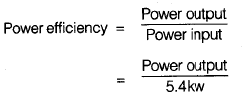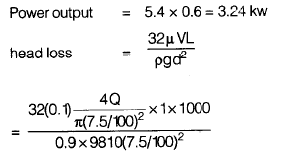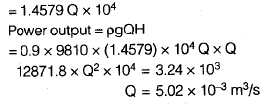## GATE Civil Engineering (CE) 2023 Mock Test Series

26 docs|292 tests
 Use Code STAYHOME200 and get INR 200 additional OFF Use Coupon Code
Information about Test: Fluid Mechanics- 1 Page
In this test you can find the Exam questions for Test: Fluid Mechanics- 1 solved & explained in the simplest way possible. Besides giving Questions and answers for Test: Fluid Mechanics- 1, EduRev gives you an ample number of Online tests for practice

## GATE Civil Engineering (CE) 2023 Mock Test Series

26 docs|292 tests